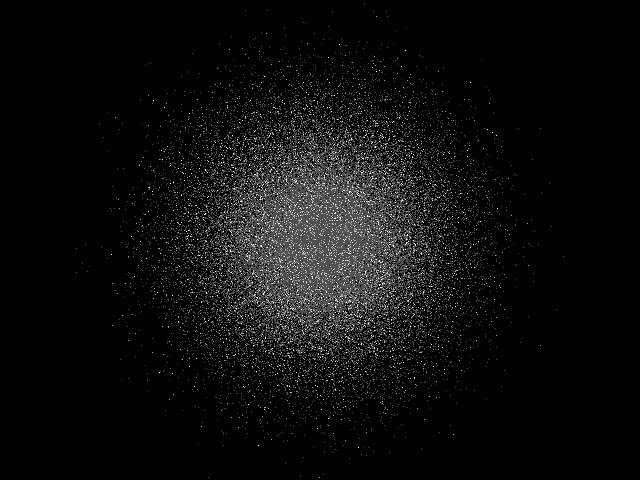### GLOBULAR CLUSTERDetails: A globular cluster is a very compact spherical group of stars. It may typically contain 100,000 to ten million stars, and is usually found in the halo of the galaxy rather than in the disc. The stars in a globular cluster are population II stars which are red and thought to be older stars deficient in elements heavier than helium. Globular clusters are typically several hundred light years in diameter. The image above is a computer simulation of a globular cluster. A random number generator is used to determine both the angular and radial position of each star in the cluster. The angle is specified by a uniform distribution, but the radial position is given by a Gaussian distribution. The simulation was done with a very simple QBASIC program whose source code is given below so that you may create your own globular clusters. ```'Generate Globular Cluster [GCLUSTER.BAS] DIM level(3) 'magnitude levels SCREEN 12 '640x480 pixels PI = 3.14159 'to put angle in radians FOR star = 1 TO 100000 '100,000 stars in cluster CLR = 8 'faintest star brightness IF star MOD 5 = 0 THEN CLR = 7 'intermediate start brightness IF star MOD 25 = 0 THEN CLR = 15 'brightest stars brightness theta = 2 * PI * RND 'select a radom angle 0 to 2pi 'now compute a radius with gaussian distribution about cluster centre radius = (RND + RND + RND + RND + RND + RND - 3!) * 100 xp = 320 + radius * COS(theta) 'compute x coordinate of star yp = 240 + radius * SIN(theta) 'compute y coordinate of star PSET (xp, yp), CLR 'and plot NEXT star DO WHILE INKEY\$ <> CHR\$(27) 'wait until ESC key is pressed LOOP END 'then terminate ``` Approximately one hundred globular clusters have been found in the halo of the milky way. The most famous in the southern sky are Omega Centauri and 47 Tucanae. These are shown below for comparison with the simulated cluster.Omega Centauri [European Southern Observatory]47 Tucanae [European Southern Observatory]